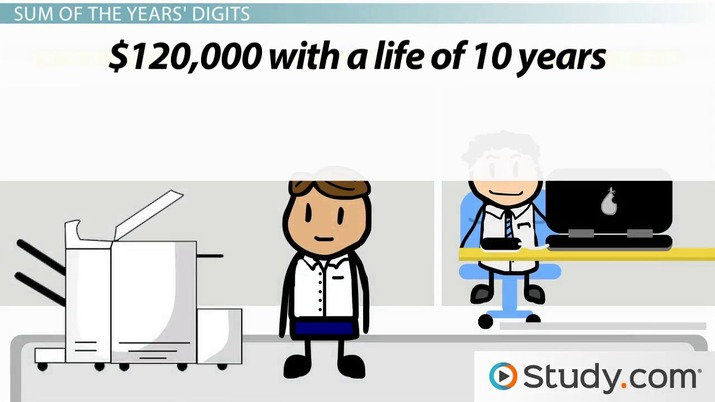# Accelerated Depreciation Methods

Lesson Transcript
Instructor: Yuanxin (Amy) Yang Alcocer

Amy has a master's degree in secondary education and has been teaching math for over 9 years. Amy has worked with students at all levels from those with special needs to those that are gifted.

Depreciation is the loss of value over time, calculated using accelerated depreciation calculations where the value is lost faster shortly after initial use, but later slows in depreciation. Learn the double declining balance method and see depreciation calculation in two examples. Updated: 10/27/2021

## Accelerated Depreciation

The basic depreciation calculation assumes that the equipment is used steadily throughout its useful life. But sometimes, you need to make accelerated depreciation calculations. This takes into account that some items depreciate more in the first few years of use, so your depreciation amounts in these years are more than later years. There are two common accelerated depreciation methods.An error occurred trying to load this video.

Try refreshing the page, or contact customer support.

Coming up next: Index Numbers in Statistics: Uses & Examples

### You're on a roll. Keep up the good work!

Replay
Your next lesson will play in 10 seconds
• 0:02 Accelerated Depreciation
• 1:10 Double Declining Balance
• 2:50 Example 1
• 3:47 Sum of the Years' Digits
• 5:35 Example 2
• 6:20 Lesson Summary
Save Save

Want to watch this again later?

Timeline
Autoplay
Autoplay
Speed Speed

## Double Declining Balance

The first method that Ron is going to talk about is the double declining balance method. This method takes your basic depreciation percentage for the year and doubles it and multiplies it by the current value of your item.

So, for example, if you were depreciating one of your large printers that cost \$120,000 with a life of ten years, the basic depreciation percent per year is 100% divided by ten years or ten percent per year. Multiplying \$120,000, the value of the item for year one by ten percent, or 0.1, gives an annual depreciation of \$12,000 for your basic depreciation for year one.

The depreciation using the double declining balance method for the first year is \$120,000 times 20%, or 0.2, double the percentage used for the basic depreciation calculation. This works out to be \$24,000 for the first year.

For year two, the printer has then dropped in value by \$24,000 since that is the depreciation you calculated the year before. Subtracting this from the original cost of \$120,000 gives \$96,000. Multiplying this \$96,000 by 20% will give the depreciation for year two. It is \$96,000 * 0.2 = \$19,200. Keep doing this until you have depreciated your value down to zero.

## Example 1

Let's look at another example. You also have this high end scanner that you bought for \$90,000. It has a lifetime of five years. Calculate the depreciation for the first two years using the double declining balance method.

A lifetime of five years gives a basic depreciation of 20% per year (100% divided by 5). Doubling this gives 40% per year for the double declining balance method. For year one, the depreciation is \$90,000 * 0.4 = \$36,000. For year two, the value drops to \$90,000 - \$36,000 = \$54,000. The depreciation for year two is \$54,000 * 0.4 = \$21,600.

## Sum of the Years' Digits

Ron now goes on to tell you that there is another method you can use to accelerate your depreciation in the first few years. This method is called sum of the years' digits. Using this method, the depreciation percentage is greatest for year one and decreases each year based on the life of the item. Ron shows you an example using the same printer that you bought for \$120,000 with a life of ten years. This method is called the sum of the years' digits because you add up the years of life that the item has: 1 + 2 + 3 + 4 + 5 + 6 + 7 + 8 + 9 + 10. This gives you a total of 55.

To unlock this lesson you must be a Study.com Member.

## Practice:Accelerated Depreciation Methods Quiz

1/5 completed

### Using the sum of the years' digits method, calculate the depreciation for year 1 of a printer purchased for \$20,000 with a life expectancy of 3 years.

Create Your Account To Take This Quiz

As a member, you'll also get unlimited access to over 84,000 lessons in math, English, science, history, and more. Plus, get practice tests, quizzes, and personalized coaching to help you succeed.

It only takes a few minutes to setup and you can cancel any time.

### Register to view this lesson

Are you a student or a teacher?